Open In App

A Spreadsheet is a computer application that is designed to add, display, analyze, organize, and manipulate data arranged in rows and columns. It is the most popular application for accounting, analytics, data presentation, etc. In other words, spreadsheets are scalable grid-based files that are used to organize data and perform calculations. People all across the world use spreadsheets to create tables for personal and business usage.

You can also use the tool’s features and formulas to help you make sense of your data.

For example – You may track data in a spreadsheet and see sums, differences, multiplication, division, and fill dates automatically, among other things. Microsoft Excel, Google Sheets, Apache Open Office, LibreOffice, etc. are some spreadsheet software. Among all these software, Microsoft Excel is the most commonly used spreadsheet tool and it is available for Windows, macOS, Android, etc.

## What is an Excel SpreadSheet?

A collection of spreadsheets is known as a workbook. Every Excel file is called a workbook. Every time you start a new project in Excel, you’ll need to create a new workbook.

There are several methods for getting started with an Excel workbook. To create a new worksheet or access an existing one, you can either start from scratch or utilize a pre-designed template. A single Excel worksheet is a tabular spreadsheet that consists of a matrix of rectangular cells grouped in rows and columns. It has a total of 1,048,576 rows and 16,384 columns, resulting in 17,179,869,184 cells on a single page of a Microsoft Excel spreadsheet where you may write, modify, and manage your data.

In the same way as a file or a book is made up of one or more worksheets that contain various types of related data, an Excel workbook is made up of one or more worksheets. You can also create and save an endless number of worksheets. The major purpose is to collect all relevant data in one place but in many categories (worksheet).

As we know that there are so many spreadsheet applications available in the market. So these applications provide the following basic features:

## Rows and columns:

Rows and columns are two distinct features in a spreadsheet that come together to make a cell, a range, or a table. In general, columns are the vertical portion of an excel worksheet, and there can be 256 of them in a worksheet, whereas rows are the horizontal portion, and there can be 1048576 of them.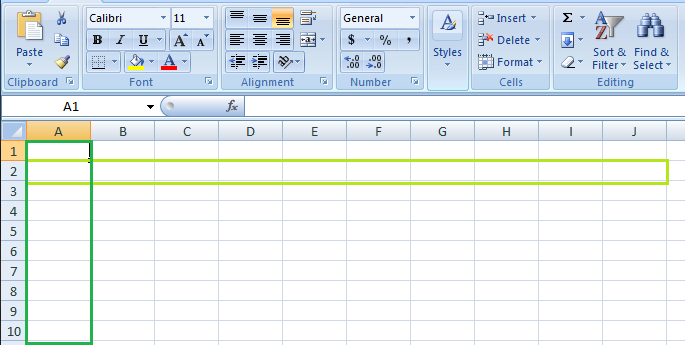The color light green is used to highlight Row 3 while the color green is used to highlight Column B. Each column has 1048576 rows and each row has 256 columns.

## Formulas:

In spreadsheets, formulas process data automatically. It takes data from the specified area of the spreadsheet as input then processes that data, and then displays the output into the new area of the spreadsheet according to where the formula is written. In Excel, we can use formulas simply by typing “=Formula Name(Arguments)” to use predefined Excel formulas. When you write the first few characters of any formula, Excel displays a drop-down menu of formulas that match that character sequence. Some of the commonly used formulas are:

• =SUM(Arg1: Arg2): It is used to find the sum of all the numeric data specified in the given range of numbers.
• =COUNT(Arg1: Arg2):  It is used to count all the number of cells(it will count only number) specified in the given range of numbers.
• =MAX(Arg1: Arg2): It is used to find the maximum number from the given range of numbers.
• =MIN(Arg1: Arg2): It is used to find the minimum number from the given range of numbers.
• =TODAY(): It is used to find today’s date.
• =SQRT(Arg1): It is used to find the square root of the specified cell.

For example, you can use the formula to find the average of the integers in column C from row 2 to row 7:

`= AVERAGE(D2:D7)`

The range of values on which you want to average is defined by D2:D6. The formula is located near the name field on the formula tab.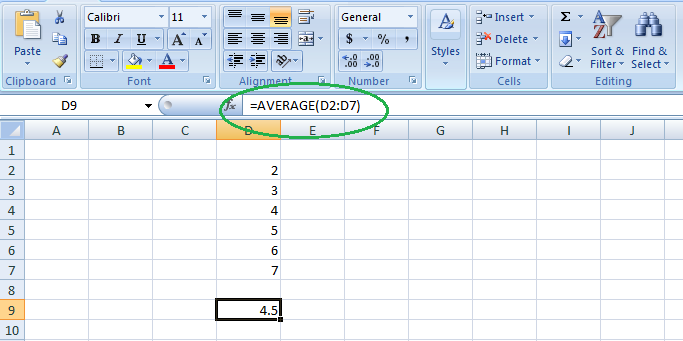We wrote =AVERAGE(D2:D6) in cell D9, therefore the average becomes (2 + 3 + 4 + 5 + 6 + 7)/6 = 27/6 = 4.5. So you can quickly create a workbook, work on it, browse through it, and save it in this manner.

In spreadsheets, the function uses a specified formula on the input and generates output. Or in other words, functions are created to perform complicated math problems in spreadsheets without using actual formulas. For example, if you want to find the total of the numeric data present in the column then use the SUM function instead of adding all the values present in the column.

## Text Manipulation:

The spreadsheet provides various types of commands to manipulate the data present in it.

Pivot Tables: It is the most commonly used feature of the spreadsheet. Using this table users can organize, group, total, or sort data using the toolbar. Or in other words, pivot tables are used to summarize lots of data. It converts tons of data into a few rows and columns.

The use of Spreadsheets is endless. It is generally used with anything that contains numbers. Some of the common use of spreadsheets are:

• Finance: Spreadsheets are used for financial data like it is used for checking account information, taxes, transaction, billing, budgets, etc.
• Forms: A spreadsheet is used to create form templates to manage performance review, timesheets, surveys, etc.
• School and colleges: Spreadsheets are most commonly used in schools and colleges to manage student’s data like their attendance, grades, etc.
• Lists: Spreadsheets are also used to create lists like grocery lists, to-do lists, contact detail, etc.
• Hotels: Spreadsheets are also used in hotels to manage the data of their customers like their personal information, room numbers, check-in date, check-out date, etc.

The basic components of spreadsheets are: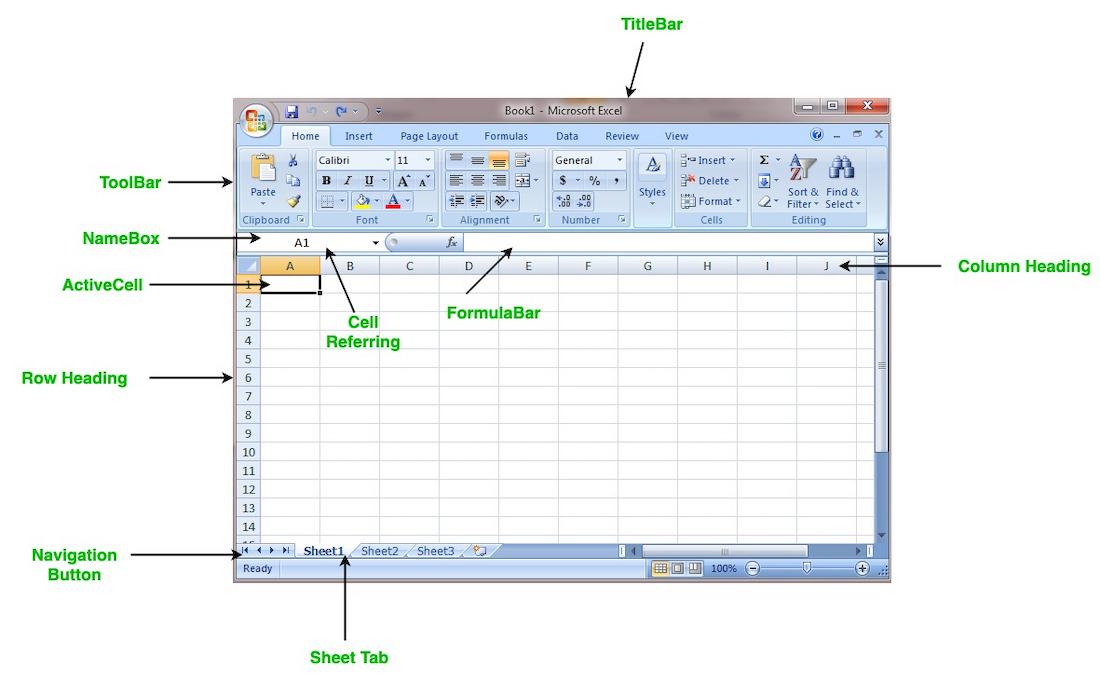### 1. TitleBar:

The title bar displays the name of the spreadsheet and application.

### 2. Toolbar:

It displays all the options or commands available in Excel for use.

### 3. NameBox:

It displays the address of the current or active cell.

### 4. Formula Bar:

It is used to display the data entered by us in the active cell. Also, this bar is used to apply formulas to the data of the spreadsheet.

Every Excel spreadsheet contains 256 columns and each column present in the spreadsheet is named by letters or a combination of letters.

Every Excel spreadsheet contains 65,536 rows and each row present in the spreadsheet is named by a number.

### 7. Cell:

In a spreadsheet, everything like a numeric value, functions, expressions, etc., is recorded in the cell. Or we can say that an intersection of rows and columns is known as a cell. Every cell has its own name or address according to its column and rows and when the cursor is present on the first cell then that cell is known as an active cell.

### 8. Cell referring:

A cell reference, also known as a cell address, is a way for describing a cell on a worksheet that combines a column letter and a row number. We can refer to any cell on the worksheet using cell references (in excel formulae). As shown in the above image the cell in column A and row 1 is referred to as A1. Such notations can be used in any formula or to duplicate the value of one cell to another (by using = A1).

A spreadsheet contains first, previous, next, and last navigation buttons. These buttons are used to move from one worksheet to another workbook.

### 10. Sheet tabs:

As we know that a workbook is a collection of worksheets. So this tab contains all the worksheets present in the workbook, by default it contains three worksheets but you can add more according to your requirement.

## How to Create a New Spreadsheet or Workbook

Step 1: Click on the top-left, Microsoft Office button and a drop-down menu appear.

Step 2: Now select New from the menu.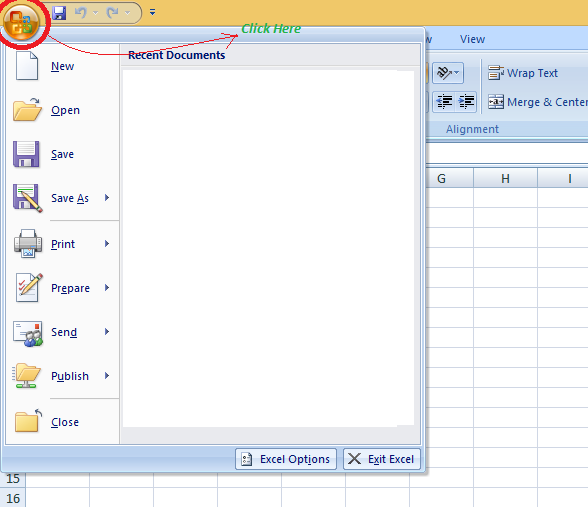Step 3: After selecting the New option a New Workbook dialogue box will appear and then in Create tab, click on the blank Document.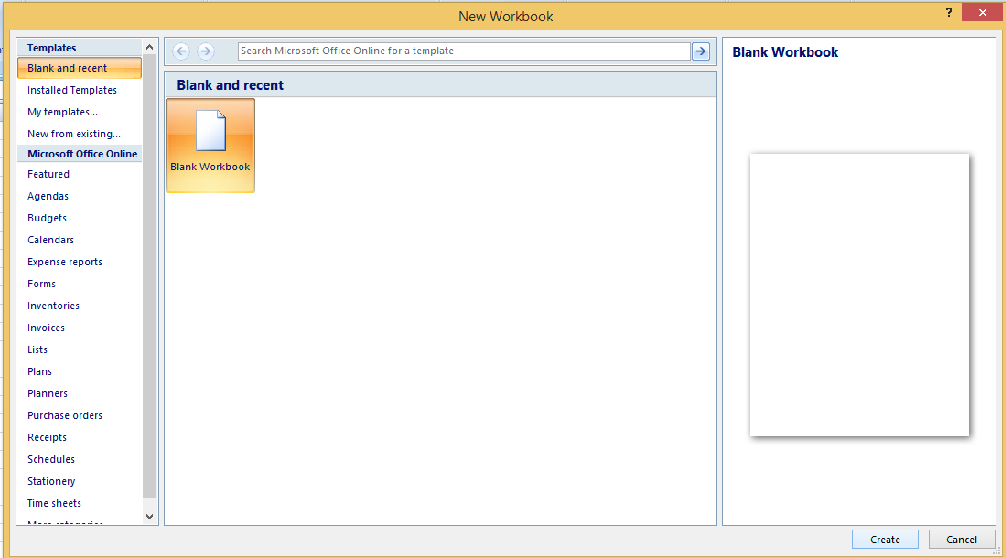A new blank worksheet is created and is shown on your screen.

Note: When you open MS Excel on your computer, it creates a new Workbook for you.

## Saving The Workbook

In Excel we can save a workbook using the following steps:

Step 1: Click on the top-left, Microsoft office button and we get a drop-down menu: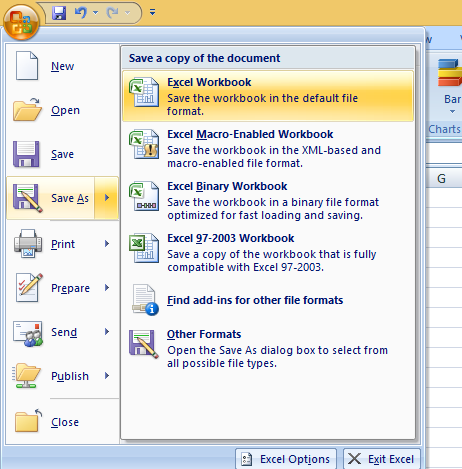Step 2: Now Save or Save As are the options to save the workbook, so choose one.

• Save As: To name the spreadsheet and then save it to a specific location. Select Save As if you wish to save the file for the first time, or if you want to save it with a new name.
• Save: To save your work, select Save/ click ctrl + S if the file has already been named.

So this is how you can save a workbook in Excel.

Excel consists of many rows and columns, each rectangular box in a row or column is referred to as a Cell. So, the combination of a column letter and a row number can be used to find a cell address on a worksheet or spreadsheet. We can refer to any cell in the worksheet using these addresses (in excel formulas). The name box on the top left(below the Home tab) displays the cell’s address whenever you click the cell.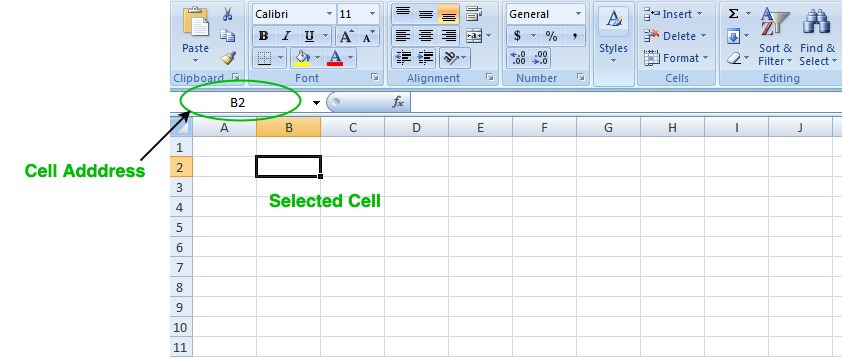To insert the data into the cell follow the following steps:

Step 1: Go to a cell and click on it

Step 2: By typing something on the keyboard, you can insert your data (In that selected cell).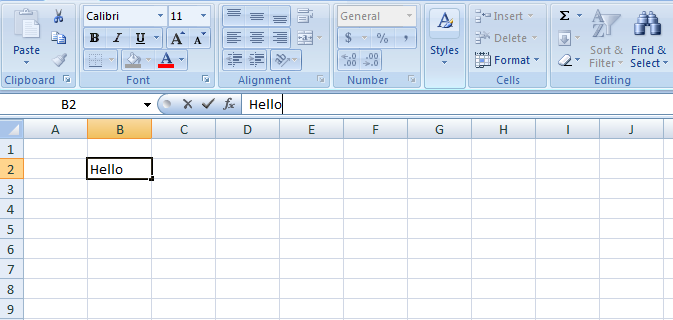Whatever text you type displays in the formula bar as well (for that cell).

## How to Edit/ Delete Cell Contents in the Spreadsheet

To delete cell content follow the following steps:

Step 1: To alter or delete the text in a cell, first select it.

Step 2: Press the Backspace key on your keyboard to delete and correct text. Alternatively, hit the Delete key to delete the whole contents of a cell. You can also edit and delete text using the formula bar. Simply select the cell and move the pointer to the formula bar.

### 1. What is SpreadSheet in Excel?

A spreadsheet is a file that exists of cells in rows and columns and can help arrange, calculate, and sort data. Data in a spreadsheet can be numeric values, as well as text, formulas, references, and functions.

### 2. Why is it called a spreadsheet?

In terms of newspapers and magazines, SpreadSheet word came into existence, i.e, it has two faces extending across the centerfold and treating the two pages as one large page.

### 3. How to create a spreadsheet?

Step 1: Open MS Excel.

Step 2: Go to Menu and select New >> Click on the Blank workbook to create a simple worksheet. OR – Press Ctrl + N: To create a new spreadsheet.

Step 3: By default, Sheet 1 will be created as a worksheet in the spreadsheet.

### 4. Who created Excel?

Charles Simonyi an Microsoft employee created Excel.